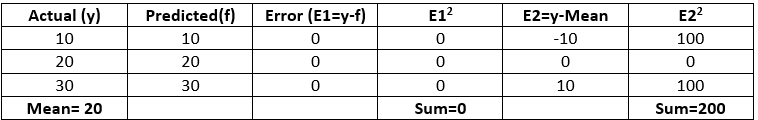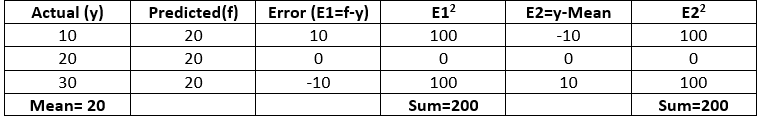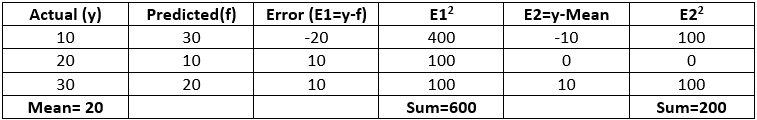Related Articles
Python – Coefficient of Determination-R2 score
• Difficulty Level : Basic
• Last Updated : 16 Jul, 2020

Coefficient of determination also called as R2 score is used to evaluate the performance of a linear regression model. It is the amount of the variation in the output dependent attribute which is predictable from the input independent variable(s). It is used to check how well-observed results are reproduced by the model, depending on the ratio of total deviation of results described by the model.

Mathematical Formula:

`R2= 1- SSres / SStot`

Where,
SSres is the sum of squares of the residual errors.
SStot is the total sum of the errors.

Interpretation of R2 score:
Assume R2 = 0.68
It can be referred that 68% of the changeability of the dependent output attribute can be explained by the model while the remaining 32 % of the variability is still unaccounted for.
R2 indicates the proportion of data points which lie within the line created by the regression equation. A higher value of R2 is desirable as it indicates better results.

Examples
Case 1 Model gives accurate results`  R2 = 1- 0/200 = 1`

Case 2 Model gives same results always` R2 = 1- 200/200 = 0`

Case 3 Model gives ambiguous results` R2 = 1- 600/200 = -2`

We can import r2_score from sklearn.metrics in Python to compute R2 score.

Python Implementation:
Code 1: Import r2_score from sklearn.metrics

 `from` `sklearn.metrics ``import` `r2_score`

Code 2: Calculate R2 score for all the above cases.

 `### Assume y is the actual value and f is the predicted values``y ``=``[``10``, ``20``, ``30``]``f ``=``[``10``, ``20``, ``30``]``r2 ``=` `r2_score(y, f)``print``(``'r2 score for perfect model is'``, r2)`

Output:

`r2 score for perfect model is 1.0`
 `### Assume y is the actual value and f is the predicted values``y ``=``[``10``, ``20``, ``30``]``f ``=``[``20``, ``20``, ``20``]``r2 ``=` `r2_score(y, f)``print``(``'r2 score for a model which predicts mean value always is'``, r2)`` `

Output:

`r2 score for a model which predicts mean value always is 0.0`

Code 3:

 `### Assume y is the actual value and f is the predicted values``y ``=` `[``10``, ``20``, ``30``]``f ``=` `[``30``, ``10``, ``20``]``r2 ``=` `r2_score(y, f)``print``(``'r2 score for a worse model is'``, r2)`

Output:

`r2 score for a worse model is -2.0`

Conclusion:

• The best possible score is 1 which is obtained when the predicted values are the same as the actual values.
• R2 score of baseline model is 0.
• During the worse cases, R2 score can even be negative.

Attention geek! Strengthen your foundations with the Python Programming Foundation Course and learn the basics.

To begin with, your interview preparations Enhance your Data Structures concepts with the Python DS Course. And to begin with your Machine Learning Journey, join the Machine Learning – Basic Level Course

My Personal Notes arrow_drop_up